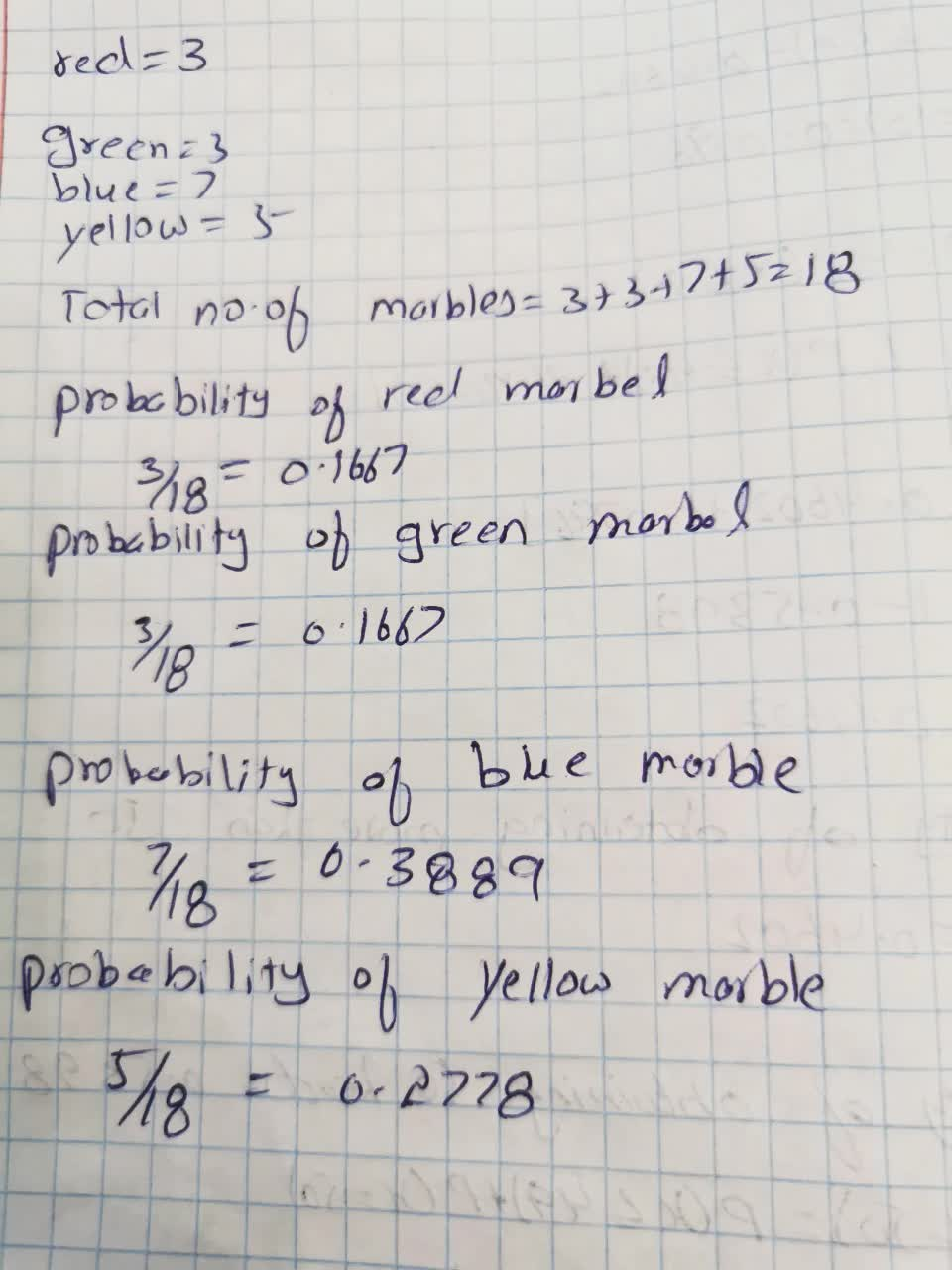# If a single marble is chosen at random from jar and it's color is recorded, give the sample space.What is the probability of each possible outcome?tricotasu 2021-09-18 Answered
A jar contains 3 red, 3 green, 7 blue, and 5 yellow marbles. If a single marble is chosen at random from the jar and it's color is recorded, give the sample space. What is the probability of each possible outcome?
You can still ask an expert for help

## Want to know more about Probability?

• Questions are typically answered in as fast as 30 minutes

Solve your problem for the price of one coffee

• Math expert for every subject
• Pay only if we can solve itpattererX
The probability is